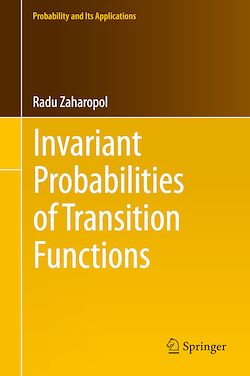# Invariant Probabilities of Transition Functions

-English
389 Pages

Description

The structure of the set of all the invariant probabilities and the structure of various types of individual invariant probabilities of a transition function are two topics of significant interest in the theory of transition functions, and are studied in this book. The results obtained are useful in ergodic theory and the theory of dynamical systems, which, in turn, can be applied in various other areas (like number theory). They are illustrated using transition functions defined by flows, semiflows, and one-parameter convolution semigroups of probability measures. In this book, all results on transition probabilities that have been published by the author between 2004 and 2008 are extended to transition functions. The proofs of the results obtained are new.

For transition functions that satisfy very general conditions the book describes an ergodic decomposition that provides relevant information on the structure of the corresponding set of invariant probabilities. Ergodic decomposition means a splitting of the state space, where the invariant ergodic probability measures play a significant role. Other topics covered include: characterizations of the supports of various types of invariant probability measures and the use of these to obtain criteria for unique ergodicity, and the proofs of two mean ergodic theorems for a certain type of transition functions.

The book will be of interest to mathematicians working in ergodic theory, dynamical systems, or the theory of Markov processes. Biologists, physicists and economists interested in interacting particle systems and rigorous mathematics will also find this book a valuable resource. Parts of it are suitable for advanced graduate courses. Prerequisites are basic notions and results on functional analysis, general topology, measure theory, the Bochner integral and some of its applications.

Subjects

##### Classwork

Informations